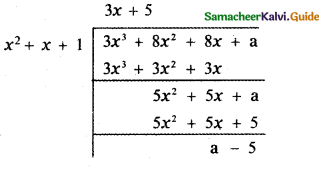Tamilnadu State Board New Syllabus Samacheer Kalvi 11th Maths Guide Pdf Chapter 2 Basic Algebra Ex 2.7 Text Book Back Questions and Answers, Notes.

## Tamilnadu Samacheer Kalvi 11th Maths Solutions Chapter 2 Basic Algebra Ex 2.7

Question 1.
Factorize x4 + 1
The given equation is x4 + 1
x4 + 1 = (x2)2 + 12
= (x2 + 1)2 – 2 (x2) (1)
[ a2 + b2 = (a + b)2 – 2ab]
= (x2 + 1)2 – (√2x)2
= (x2 + 1 + √2x) (x2 + 1 – √2x)
x4 + 1 = (x2 + 2x + 1) (x2 – √2x + 1)Question 2.
If x4 + x + 1 is a factor of the polynomial 3x3 + 8x2 + 8x + a, then find the value of a.